[top.htm]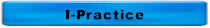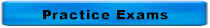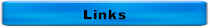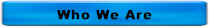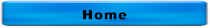Answers to More Distribution Questions

The purpose of the USEPA Ground Water Rule is to reduce disease incidence associated with disease-causing microorganisms in

A.  Drinking water
B.  Non-point sources
C.  Storm water
D.  Wastewater

Source:  USEPA Ground Water Rule, http://www.epa.gov/safewater/disinfection/gwr/regulation.html

The correct answer is A.  Drinking Water

The USEPA Groundwater Rule becomes effective on

A. December 1, 2009

B. July 1, 2010

C. December 1, 2010

D. July 1, 2011

Source:  Iowa Department of Natural Resources' Water Supply Listserv - November 10, 2009

The correct answer is A.  December 1, 2009

What is the minimum recommended pressure in a water distribution system? What is this equivalent to in feet of head?

Minimum pressure is 20 psi

20 psi x 2.31 ft/psi = 46.2 ft of head

Chlorine ton cylinders at room temperature will deliver gas at a maximum rate of

150 lb cylinders        Ton Containers

A.  10 pounds per day      100 pounds per day

B.  25 pounds per day      250 pounds per day

C.  40 pounds per day      450 pounds per day

D.  150 pounds per day    2000 pounds per day

The correct answer is C

Which of the following valves only permits flow to move in one direction?

A.  Butterfly

B.  Check

C.  Gate

D.  Plug

The correct answer is B

The specific gravity of chlorine gas is

A.  1

B.  Greater than 1

C.  Less than 1

The correct answer is B

 Matching 1.    Calcium A.  C 2.    Carbon B.  S 3.    Chlorine C.  P 4.    Copper D.  K 5.    Fluorine E.  O 6.    Iron F.   Na 7.    Hydrogen G.  N 8.    Lead H.  Mg 9.    Magnesium I.    Ca 10.  Manganese J.   Cl 11.  Nitrogen K.  Cu 12.  Potassium L.   Mn 13.  Sodium M.   Fe 14.  Oxygen N.   H 15.  Phosphorus O.   Pb 16.  Sulfur P.   Fl

1.  I

2.  A

3.  J

4.  K

5.  P

6.  M

7.  N

8.  O

9.  H

10.  L

11.  G

12.  D

13.  F

14.  E

15.  C

16.  B

Which of the following is correct?

A.  A is the follower and B is the lantern ring

B.  A is the lantern ring and B is a packing ring

C.  A is a packing ring and B is the lantern ring

D.  A is a packing ring and B is the follower

The correct answer is C.  A is a packing ring and B is the lantern ring

pH measures

A.  H+

B.  OH-

C.  O2

D.  Phosphorus

The correct answer is A.  H+  -- pH measures the hydrogen ion concentration

How do you know that your pump is cavitating?

A.  It doesn't sound right

B.  It runs hot

C.  It won't start

D.   All of the above

The correct answer is A.  It doesn't sound right.

A pump that's cavitating sounds like it's pumps rocks or gravel.

A chlorine atom is composed of

A.  Electrons

B.  Neutrons

C.  Protons

D.  All of the above

The correct answer is D.  All of the Above

Throttling the discharge valve on a centrifugal pump will the likely ______ temperature of the motor and ______ the temperature of the pump

A.  Increase, increase

B.  Increase, reduce

C.  Reduce, increase

D.  Reduce, reduce

The correct answer is C.  Reduce, increase

A, B, and D are incorrect because throttling the discharge valve on a centrifugal pump will the likely reduce temperature of the motor and increase the temperature of the pump

The chemical symbol for chlorine gas is

A.  Cl

B.  Cl-

C.  Cl2

D.  HOCl

The correct answer is C.  Cl2

A is incorrect because this is chemical symbol for chlorine not chlorine gas

B is incorrect because this is the chemical symbol for chloride

D is incorrect because this is the chemical formula for hypochlorite

Which of the following is the indicator used for pathogens?

A.  Amoebic dysentery

B.  E. coli

C.  Salmonella typhosa

D.  V. comma

The correct answer is B.  E. coli

A is incorrect because this is a pathogen, not an indicator

C is incorrect because this is a pathogen (cholera), not an indicator

D is incorrect because this is a pathogen (typhoid), not an indicator

Pump suction and discharge pressures are usually measured in

A.  gpm

B.  kWh

C.  mg/L

D.  psi

The correct answer is D.  psi

A is incorrect because this is a measure of flow

B is incorrect because this is a measure of energy used

C is incorrect because this is a measure of concentration

Which of the following is the correct formula for chlorine in water?

A.  Cl2 + H2O → HOCl + HCl

B.  NaOCl + H2O → HOCl + NaOH

C.  Ca(OCl)2 + 2H2O → 2HOCl + Ca(OH)2

D.  HOCl → H+ + OCl-

E.  All of the above

The correct answer is E.  All of the Above

The PE for flow is _____ gpcd.

A.  7.48

B.  8.34

C.  150

D.  250

The correct answer is C.  150 gpcd

A is incorrect because this is the conversion factor from cubic foot to gallon

B is incorrect because this is the conversion factor between gallon and pounds

D is incorrect because a breakdown in the disinfection process would lead to high levels of pathogens

For questions or comments, call 515-313-1159

Home